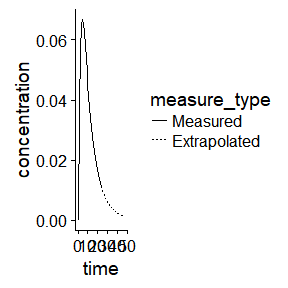# Introduction

Area under the curve (AUC) has many versions, and subtleties in differences between AUC types can lead to selecting the correct (or incorrect) one for an analysis.

single_dose_oral <- function(t=0:24, F=1, D=1, ka=0.5, Vd=10, ke=0.01) {
F*D*ka/(Vd*(ka-ke))*(exp(-ke*t) - exp(-ka*t))
}

simdose <- data_frame(time=0:48,
ke=0.1,
half.life=log(2)/ke,
concentration=single_dose_oral(t=time, ke=ke),
measure_type=factor(
case_when(time <= 24~"Measured",
time > 24~"Extrapolated"),
levels=c("Measured", "Extrapolated")))
simdose <-
bind_rows(simdose,
simdose %>%
filter(time == 24) %>%
mutate(measure_type=factor("Extrapolated",
levels=levels(simdose\$measure_type)))) %>%
arrange(time, measure_type)

ggplot(simdose, aes(x=time, y=concentration, linetype=measure_type)) +
geom_line()For the above concentration-time curve